# 七、分割技术

• 阈值技术简介
• 简单阈值技术
• 自适应阈值技术
• 大津阈值算法
• 三角阈值算法
• 彩色图像的阈值
• 使用 scikit-image 的阈值算法

# 技术要求

• Python 和 OpenCV。
• 特定于 Python 的 IDE。
• NumPy 和 Matplotlib 包。
• scikit-image 图像处理库（对于本章的最后部分是可选的。请参阅 scikit-image 阈值算法以了解如何为基于 Conda 的发行版安装它）。 请参阅以下说明，以便使用pip进行安装。
• 还需要 SciPy 库（对于本章的最后部分是可选的）。 请参阅以下说明，以便使用pip进行安装。
• 一个 Git 客户端。

$pip install scikit-image  或者，您也可以为基于 Conda 的发行版安装 scikit-image，如使用该库的特定部分所述。 要检查安装是否正确执行，只需打开 Python shell 并尝试按以下方式导入scikit-image库： python import skimage  # 安装 SciPy 要安装 SciPy，请使用以下命令： $ pip install scipy


python
import scipy


# 阈值技术简介

OpenCV 提供cv2.threshold()函数以对图像进行阈值处理。 我们将在本章接下来的小节中详细介绍该函数。

thresholding_introduction.py脚本中，我们将cv2.threshold()函数与一些预定义的阈值一起应用-050100150200250，以便查看对于不同的阈值图像如何变化。

ret1, thresh1 = cv2.threshold(gray_image, 50, 255, cv2.THRESH_BINARY)


ret5, thresh5 = cv2.threshold(gray_image, 200, 255, cv2.THRESH_BINARY)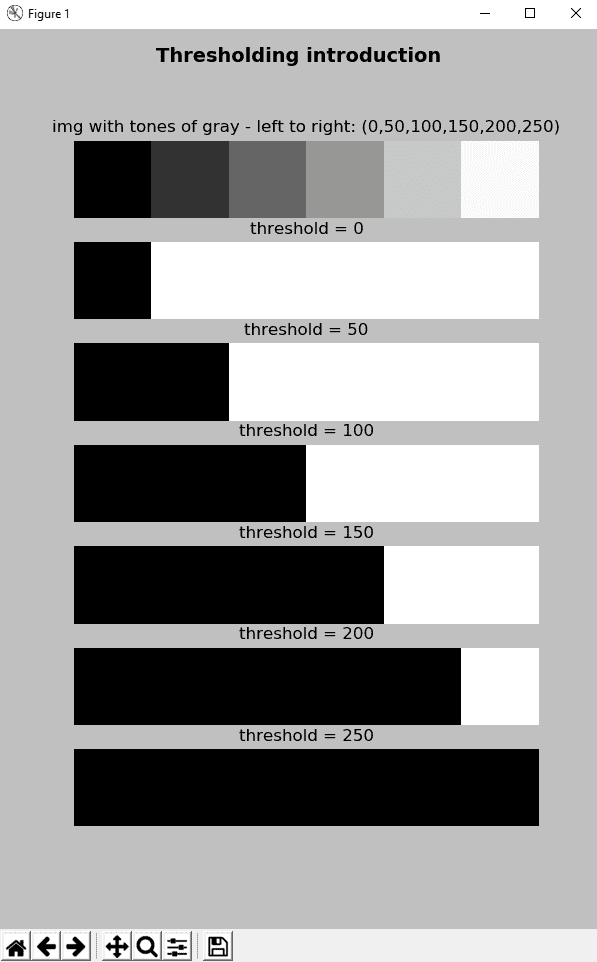def build_sample_image():
"""Builds a sample image with 50x50 regions of different tones of gray"""

# Define the different tones.
# The end of interval is not included
tones = np.arange(start=50, stop=300, step=50)
# print(tones)

# Initialize result with the first 50x50 region with 0-intensity level
result = np.zeros((50, 50, 3), dtype="uint8")

# Build the image concatenating horizontally the regions:
for tone in tones:
img = np.ones((50, 50, 3), dtype="uint8") * tone
result = np.concatenate((result, img), axis=1)

return result


• np.ones()：这将返回给定形状和类型的数组，并填充为 1； 在这种情况下，形状为(50, 50, 3)dtype="uint8"
• np.zeros()：这将返回给定形状和类型的数组，并用零填充； 在这种情况下，形状为(50, 50, 3)dtype="uint8"
• np.arange()：考虑到提供的步骤，它会在给定的间隔内返回均匀间隔的值。 不包括间隔的末尾（在这种情况下为300）。
• np.concatenate()：这将沿着现有轴（在本例中为axis=1）连接一系列数组，以水平连接图像。

ret1, thresh1 = cv2.threshold(gray_image, 0, 255, cv2.THRESH_BINARY)
ret2, thresh2 = cv2.threshold(gray_image, 50, 255, cv2.THRESH_BINARY)
ret3, thresh3 = cv2.threshold(gray_image, 100, 255, cv2.THRESH_BINARY)
ret4, thresh4 = cv2.threshold(gray_image, 150, 255, cv2.THRESH_BINARY)
ret5, thresh5 = cv2.threshold(gray_image, 200, 255, cv2.THRESH_BINARY)
ret6, thresh6 = cv2.threshold(gray_image, 250, 255, cv2.THRESH_BINARY)


# 简单阈值

cv2.threshold(src, thresh, maxval, type, dst=None) -> retval, dst


cv2.threshold()函数将固定级别的阈值应用于src输入数组（多通道，8 位或 32 位浮点）。 固定级别由thresh参数调整，该参数设置阈值。 type参数设置阈值类型，这将在下一个小节中进一步说明。

• cv2.THRESH_BINARY
• cv2.THRESH_BINARY_INV
• cv2.THRESH_TRUNC
• cv2.THRESH_TOZERO
• cv2.THRESH_TOZERO_INV
• cv2.THRESH_OTSU
• cv2.THRESH_TRIANGLE

# 阈值类型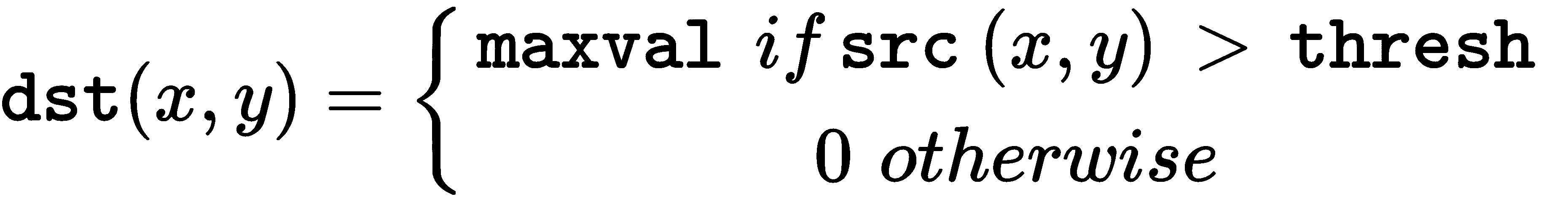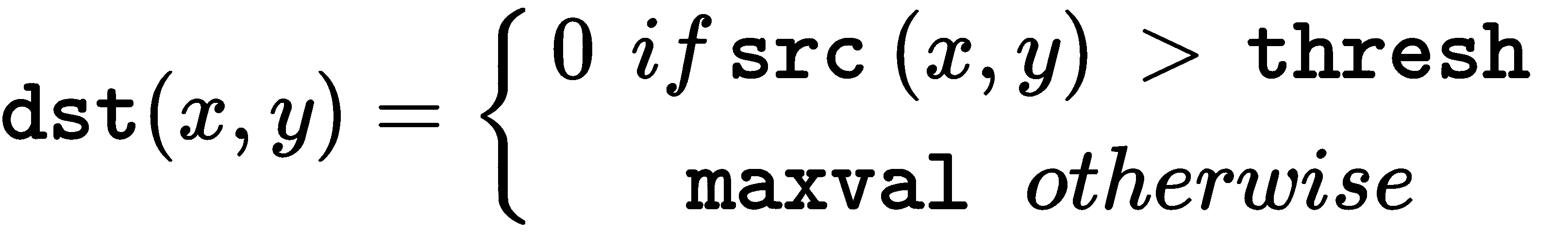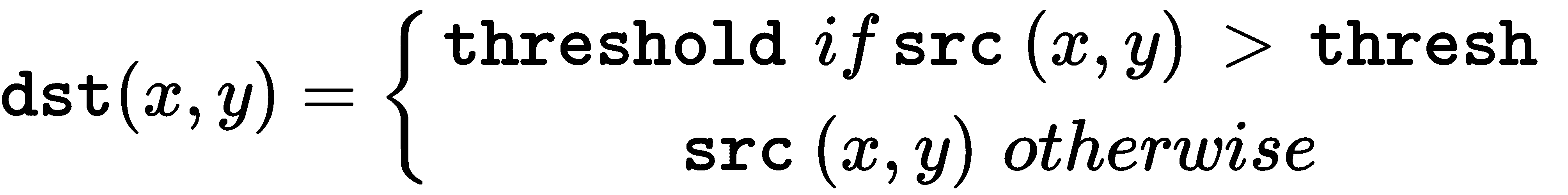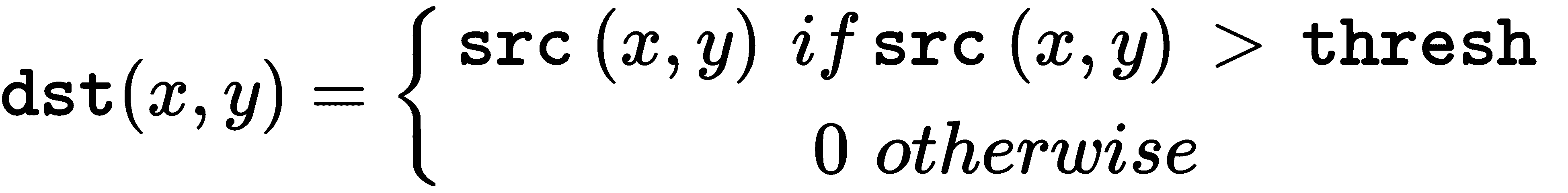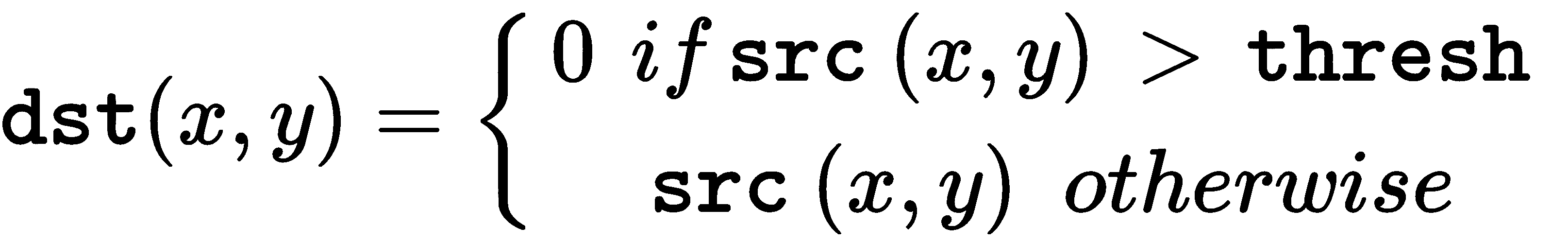thresholding_simple_types.py脚本可帮助您了解上述类型。 我们使用上一节中介绍的相同样本图像，并对所有先前类型使用固定阈值（thresh = 100）执行阈值操作。

ret1, thresh1 = cv2.threshold(gray_image, 100, 255, cv2.THRESH_BINARY)
ret2, thresh2 = cv2.threshold(gray_image, 100, 220, cv2.THRESH_BINARY)
ret3, thresh3 = cv2.threshold(gray_image, 100, 255, cv2.THRESH_BINARY_INV)
ret4, thresh4 = cv2.threshold(gray_image, 100, 220, cv2.THRESH_BINARY_INV)
ret5, thresh5 = cv2.threshold(gray_image, 100, 255, cv2.THRESH_TRUNC)
ret6, thresh6 = cv2.threshold(gray_image, 100, 255, cv2.THRESH_TOZERO)
ret7, thresh7 = cv2.threshold(gray_image, 100, 255, cv2.THRESH_TOZERO_INV)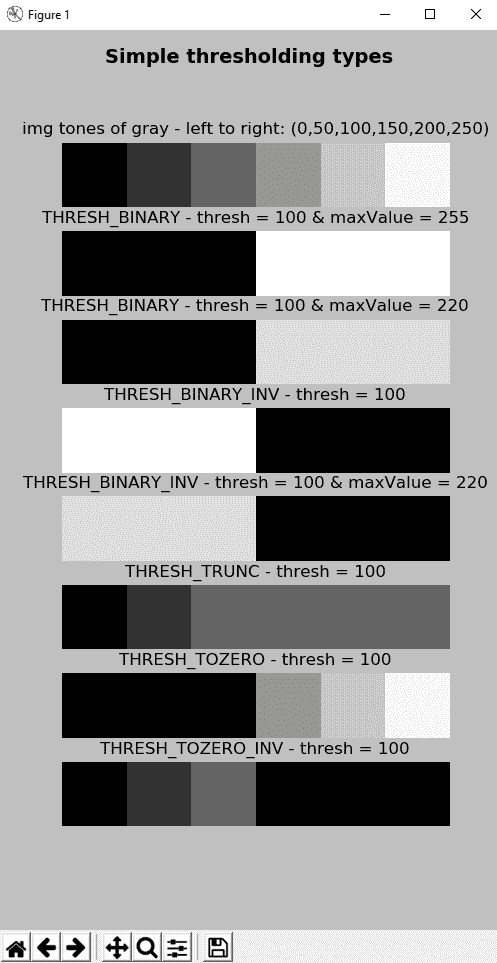# 应用于真实图像的简单阈值

ret1, thresh1 = cv2.threshold(gray_image, 60, 255, cv2.THRESH_BINARY)
ret2, thresh2 = cv2.threshold(gray_image, 70, 255, cv2.THRESH_BINARY)
ret3, thresh3 = cv2.threshold(gray_image, 80, 255, cv2.THRESH_BINARY)
ret4, thresh4 = cv2.threshold(gray_image, 90, 255, cv2.THRESH_BINARY)
ret5, thresh5 = cv2.threshold(gray_image, 100, 255, cv2.THRESH_BINARY)
ret6, thresh6 = cv2.threshold(gray_image, 110, 255, cv2.THRESH_BINARY)
ret7, thresh7 = cv2.threshold(gray_image, 120, 255, cv2.THRESH_BINARY)
ret8, thresh8 = cv2.threshold(gray_image, 130, 255, cv2.THRESH_BINARY)


show_img_with_matplotlib(cv2.cvtColor(thresh1, cv2.COLOR_GRAY2BGR), "threshold = 60", 2)
show_img_with_matplotlib(cv2.cvtColor(thresh2, cv2.COLOR_GRAY2BGR), "threshold = 70", 3)
show_img_with_matplotlib(cv2.cvtColor(thresh3, cv2.COLOR_GRAY2BGR), "threshold = 80", 4)
show_img_with_matplotlib(cv2.cvtColor(thresh4, cv2.COLOR_GRAY2BGR), "threshold = 90", 5)
show_img_with_matplotlib(cv2.cvtColor(thresh5, cv2.COLOR_GRAY2BGR), "threshold = 100", 6)
show_img_with_matplotlib(cv2.cvtColor(thresh6, cv2.COLOR_GRAY2BGR), "threshold = 110", 7)
show_img_with_matplotlib(cv2.cvtColor(thresh7, cv2.COLOR_GRAY2BGR), "threshold = 120", 8)
show_img_with_matplotlib(cv2.cvtColor(thresh8, cv2.COLOR_GRAY2BGR), "threshold = 130", 9)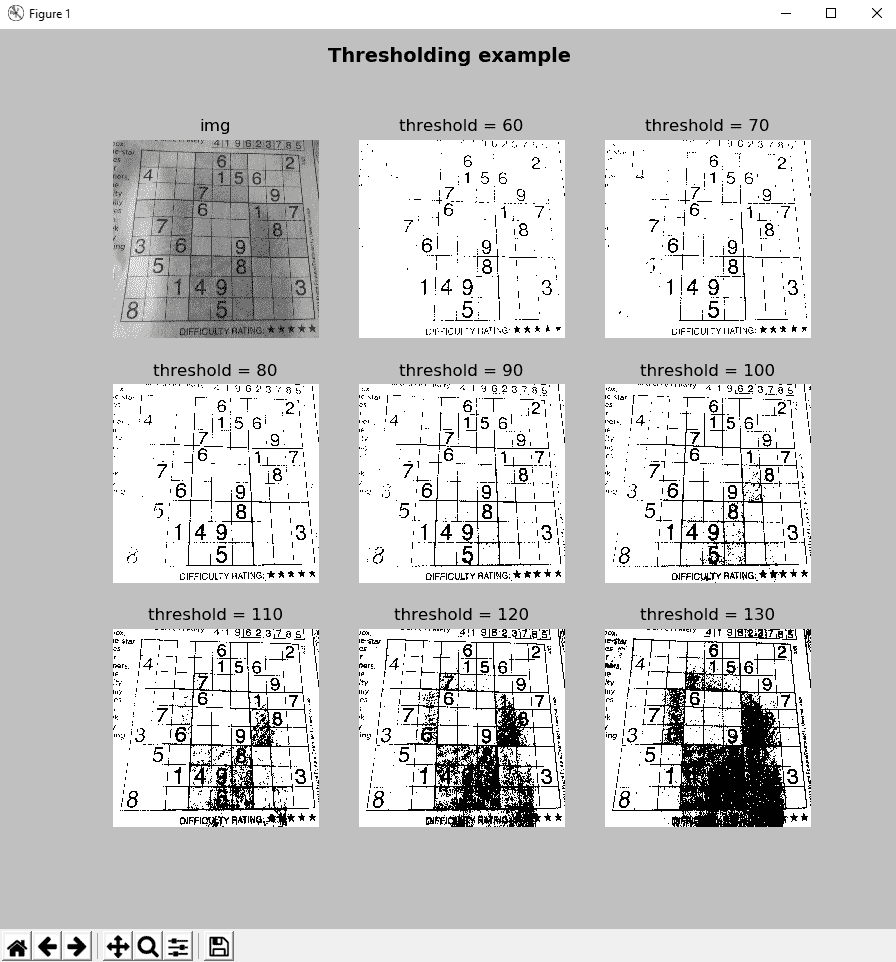# 自适应阈值

adaptiveThreshold(src, maxValue, adaptiveMethod, thresholdType, blockSize, C[, dst]) -> dst


• cv2.ADAPTIVE_THRESH_MEAN_CT(x, y)阈值计算为(x, y)blockSize x blockSize邻域平均值减去C参数
• cv2.ADAPTIVE_THRESH_GAUSSIAN_C：将T(x, y)阈值计算为(x, y)blockSize x blockSize邻域的加权总和减去C参数

blockSize参数设置用于计算像素阈值的邻域的大小，并且可以采用3, 5, 7,...等值。

C参数只是从均值或加权均值中减去的常数（取决于adaptiveMethod参数设置的自适应方法）。 通常，此值为正，但可以为零或负。 最后，thresholdType 参数设置cv2.THRESH_BINARYcv2.THRESH_BINARY_INV阈值类型。

• 这是cv2.THRESH_BINARY的公式：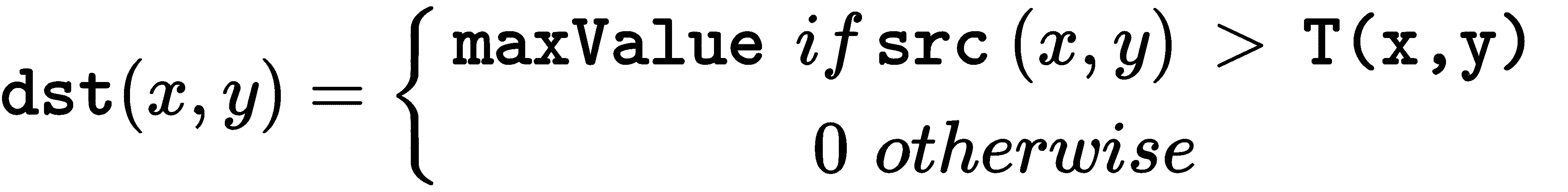• 这是cv2.THRESH_BINARY_INV的公式：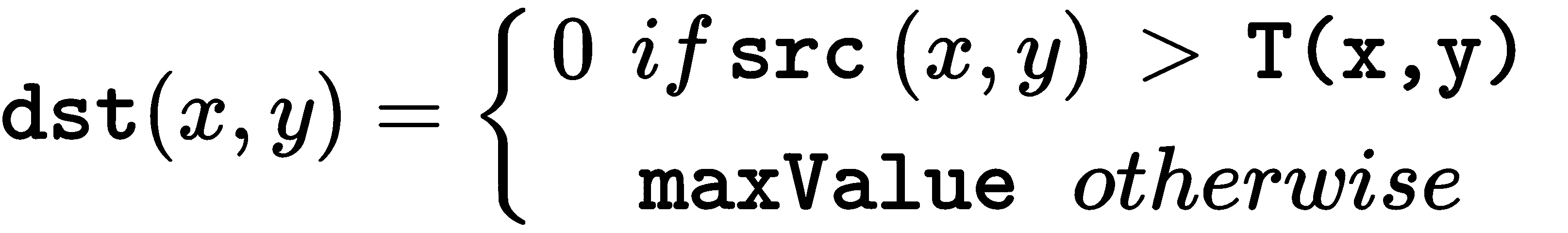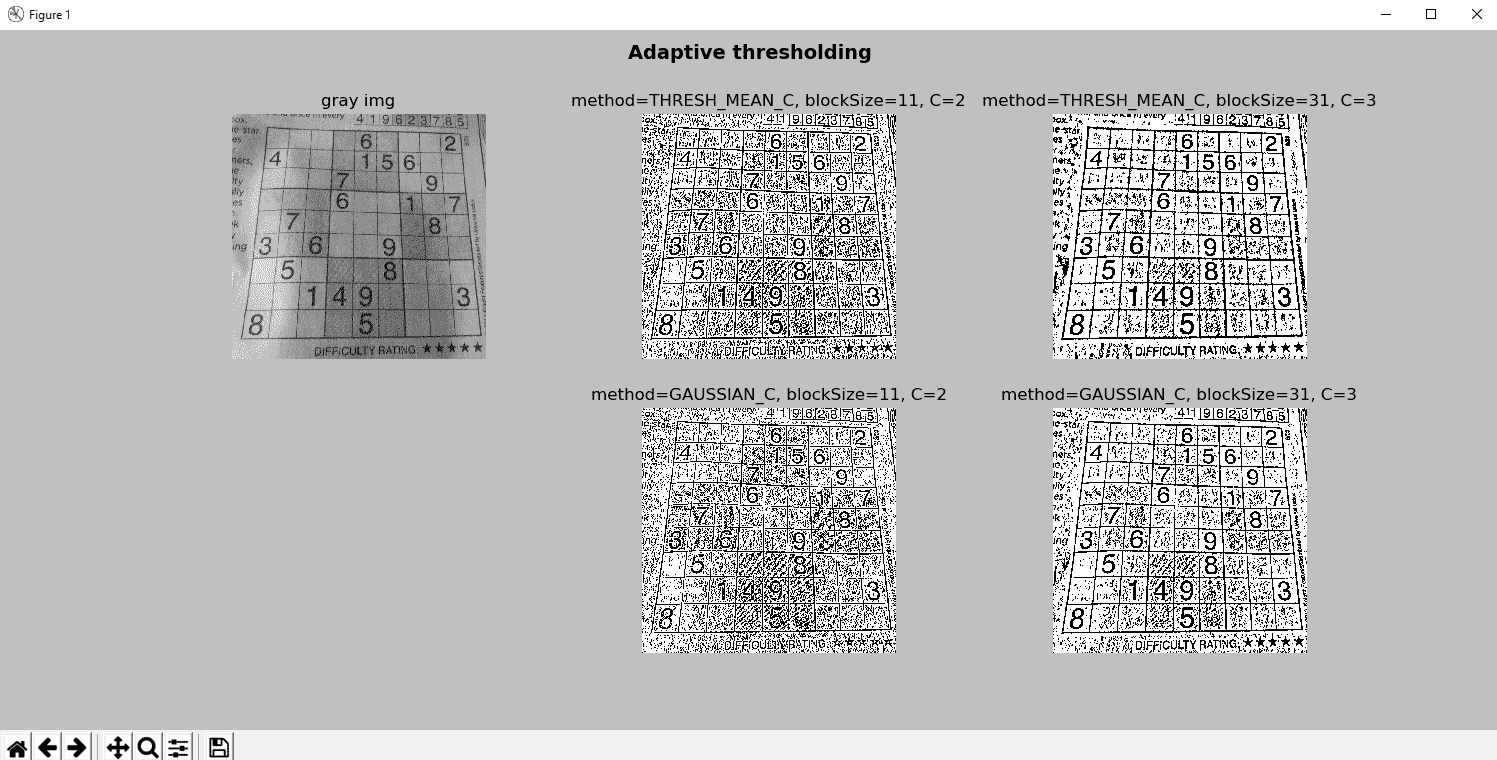gray_image = cv2.bilateralFilter(gray_image, 15, 25, 25)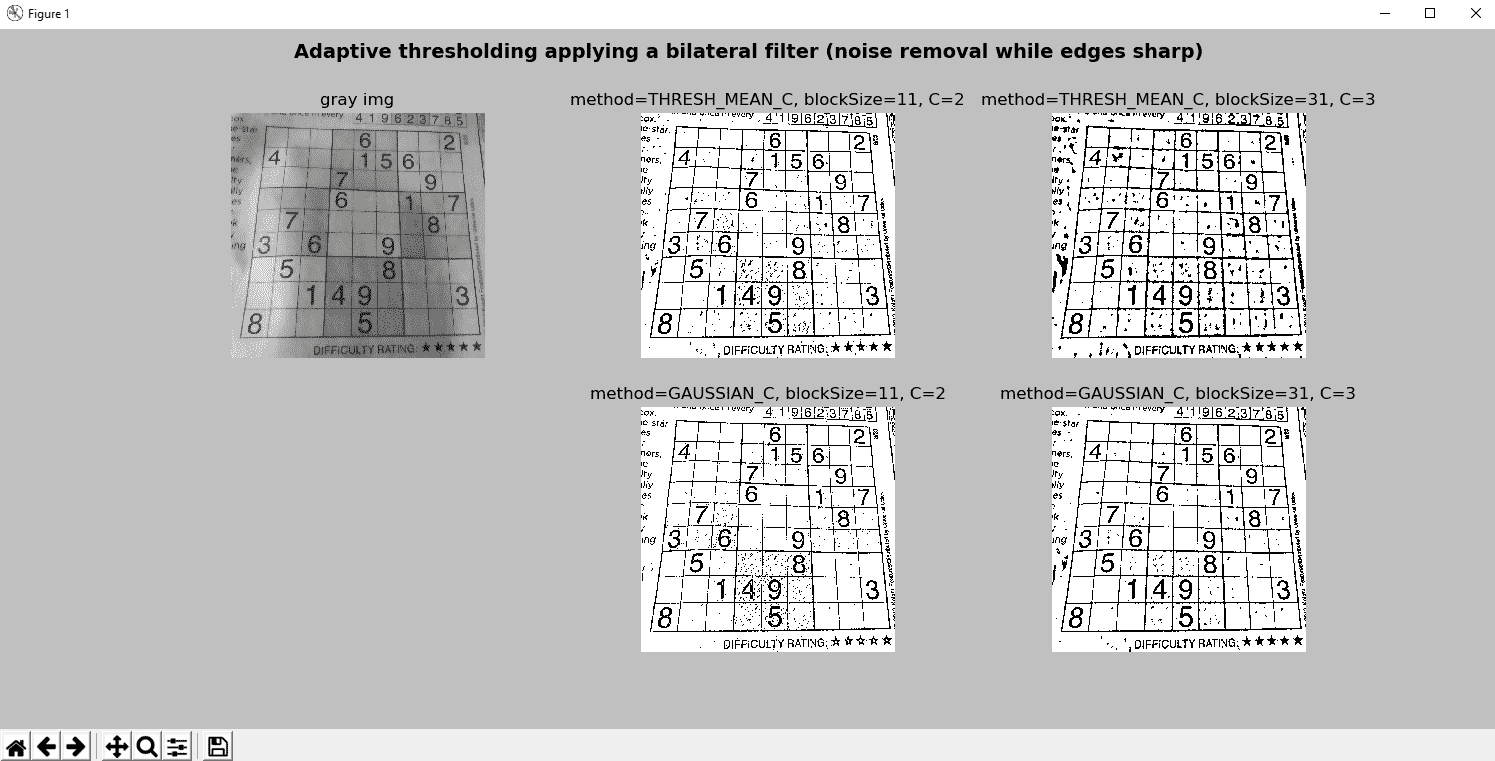# 大津阈值算法

ret, th = cv2.threshold(gray_image, 0, 255, cv2.THRESH_BINARY + cv2.THRESH_OTSU)


thresholding_otsu.py脚本中，我们已将此算法应用于样本图像。 在以下屏幕截图中可以看到输出。 我们修改了show_hist_with_matplotlib_gray()函数，添加了一个额外的参数，该参数对应于大津算法计算出的最佳阈值。 为了绘制此阈值，我们绘制一条线，以t阈值建立x坐标，如下所示：

plt.axvline(x=t, color='m', linestyle='--')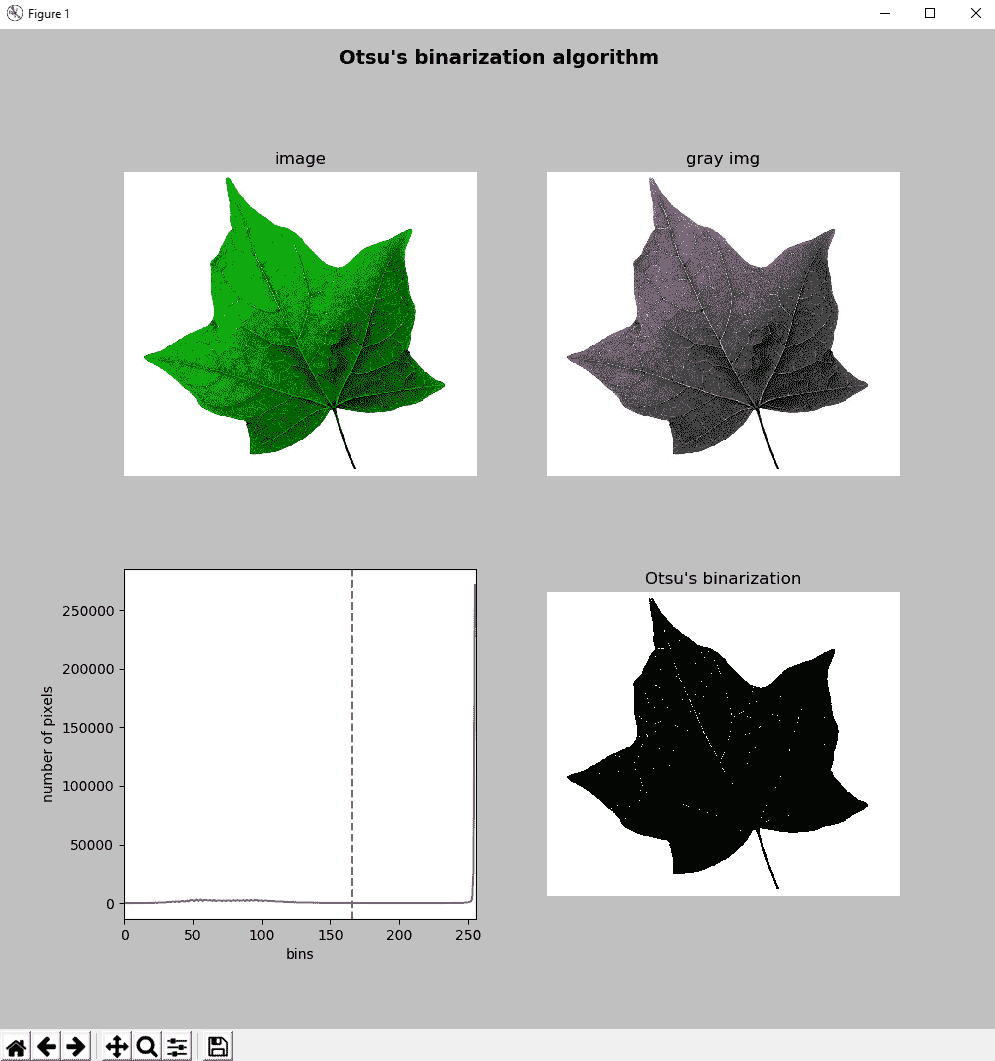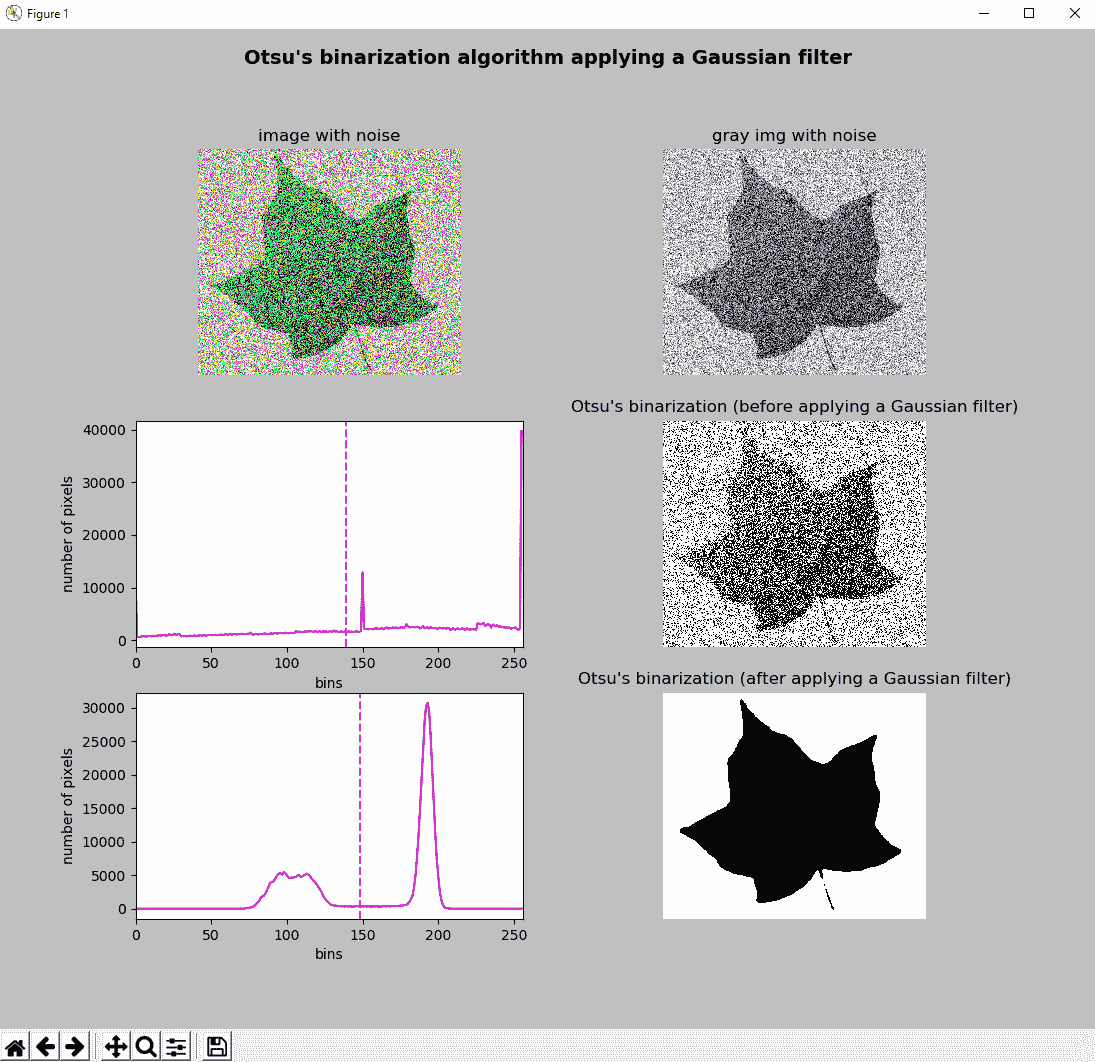# 三角二值化算法

ret1, th1 = cv2.threshold(gray_image, 0, 255, cv2.THRESH_BINARY + cv2.THRESH_TRIANGLE)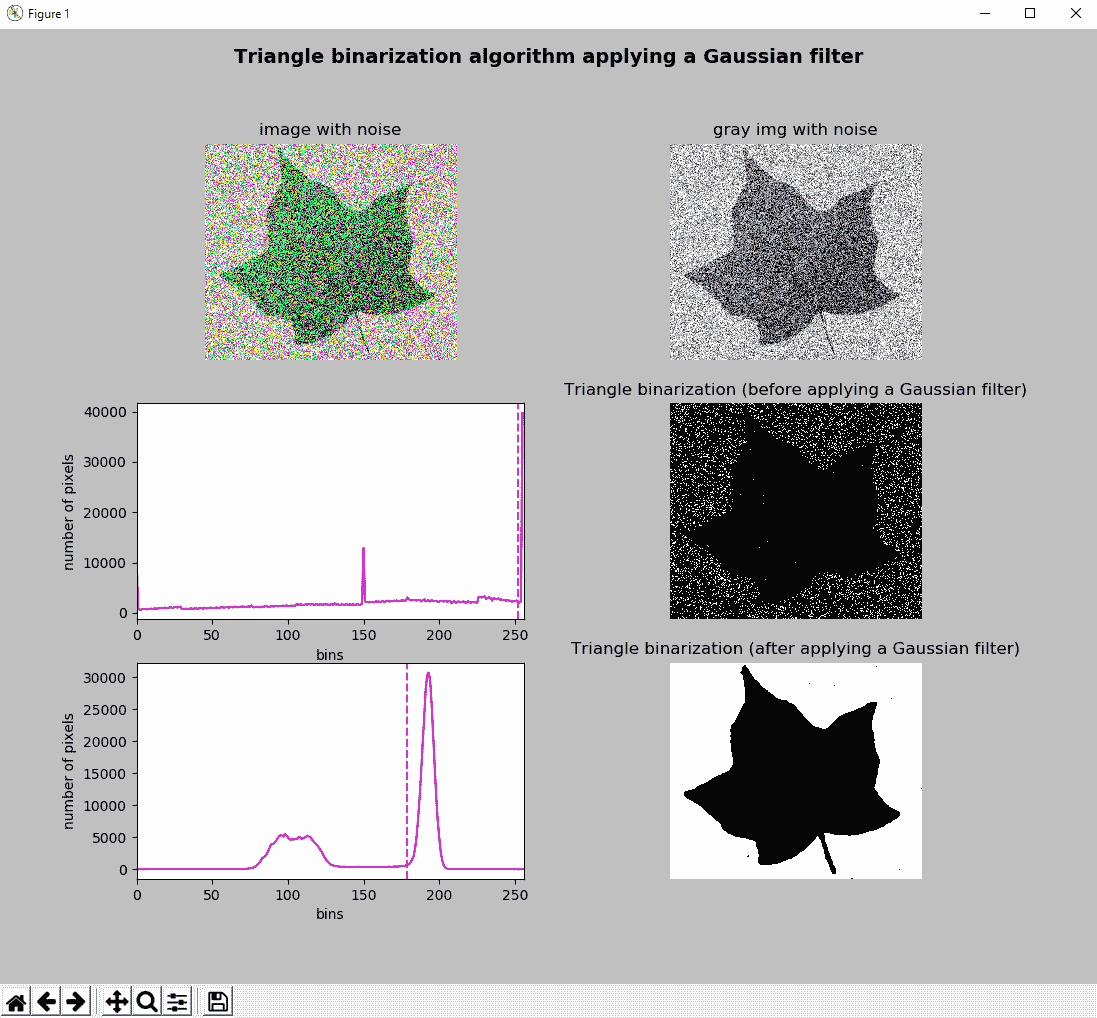# 彩色图像的阈值

cv2.threshold()函数也可以应用于多通道图像。 可以在thresholding_bgr.py脚本中看到。 在这种情况下，cv2.threshold()函数在 BGR 图像的每个通道中应用阈值操作。 这产生与在每个通道中应用此函数并合并阈值通道相同的结果：

ret1, thresh1 = cv2.threshold(image, 150, 255, cv2.THRESH_BINARY)


(b, g, r) = cv2.split(image)
ret2, thresh2 = cv2.threshold(b, 150, 255, cv2.THRESH_BINARY)
ret3, thresh3 = cv2.threshold(g, 150, 255, cv2.THRESH_BINARY)
ret4, thresh4 = cv2.threshold(r, 150, 255, cv2.THRESH_BINARY)
bgr_thresh = cv2.merge((thresh2, thresh3, thresh4))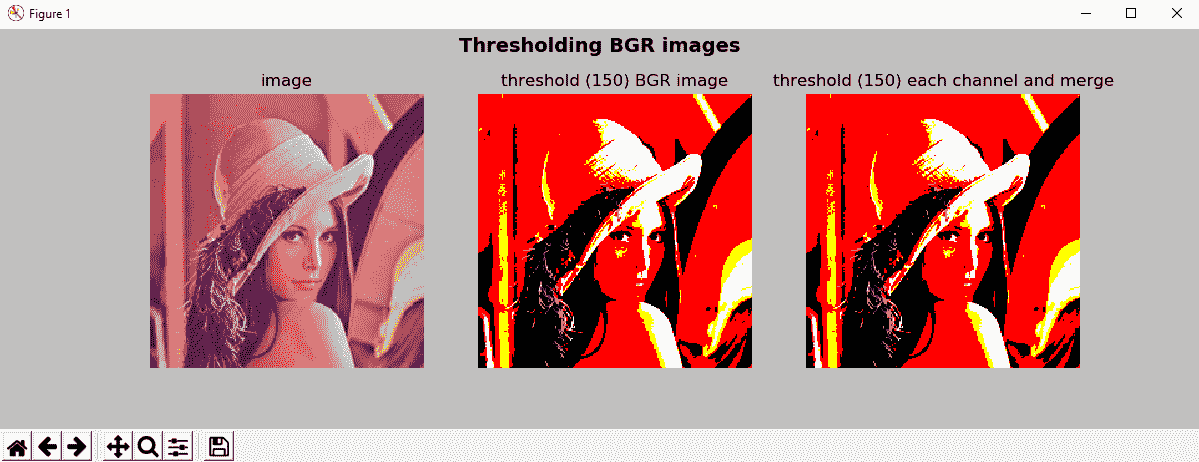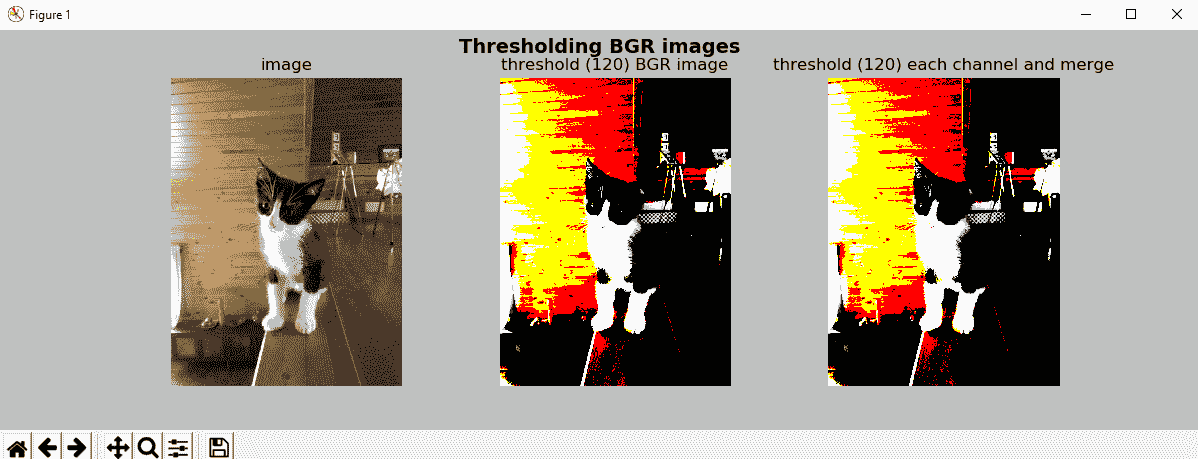# 使用 scikit-image 的阈值算法

conda install -c conda-forge scikit-image


# 使用 scikit-image 介绍阈值

from skimage.filters import threshold_otsu
from skimage import img_as_ubyte


thresh = threshold_otsu(gray_image)
binary = gray_image > thresh
binary = img_as_ubyte(binary)


threshold_otsu(gray_image)函数基于大津的二值化算法返回阈值。 然后，使用该值构造二进制图像（dtype= bool），应将其转换为 8 位无符号整数格式（dtype= uint8）以进行适当的可视化。 img_as_ubyte()函数用于此目的。 该示例的完整代码可以在thresholding_scikit_image_otsu.py脚本中看到。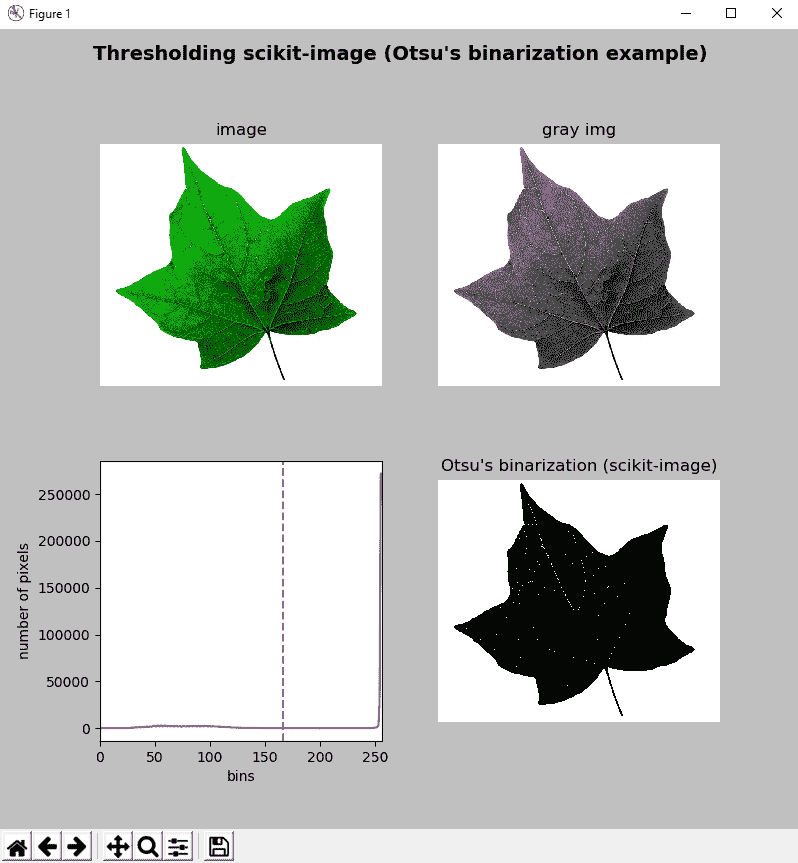# 尝试使用 scikit-image 更多的阈值技术

from skimage.filters import (threshold_otsu, threshold_triangle, threshold_niblack, threshold_sauvola)
from skimage import img_as_ubyte


# Trying Otsu's scikit-image algorithm:
thresh_otsu = threshold_otsu(gray_image)
binary_otsu = gray_image > thresh_otsu
binary_otsu = img_as_ubyte(binary_otsu)

# Trying Niblack's scikit-image algorithm:
thresh_niblack = threshold_niblack(gray_image, window_size=25, k=0.8)
binary_niblack = gray_image > thresh_niblack
binary_niblack = img_as_ubyte(binary_niblack)

# Trying Sauvola's scikit-image algorithm:
thresh_sauvola = threshold_sauvola(gray_image, window_size=25)
binary_sauvola = gray_image > thresh_sauvola
binary_sauvola = img_as_ubyte(binary_sauvola)

# Trying triangle scikit-image algorithm:
thresh_triangle = threshold_triangle(gray_image)
binary_triangle = gray_image > thresh_triangle
binary_triangle = img_as_ubyte(binary_triangle)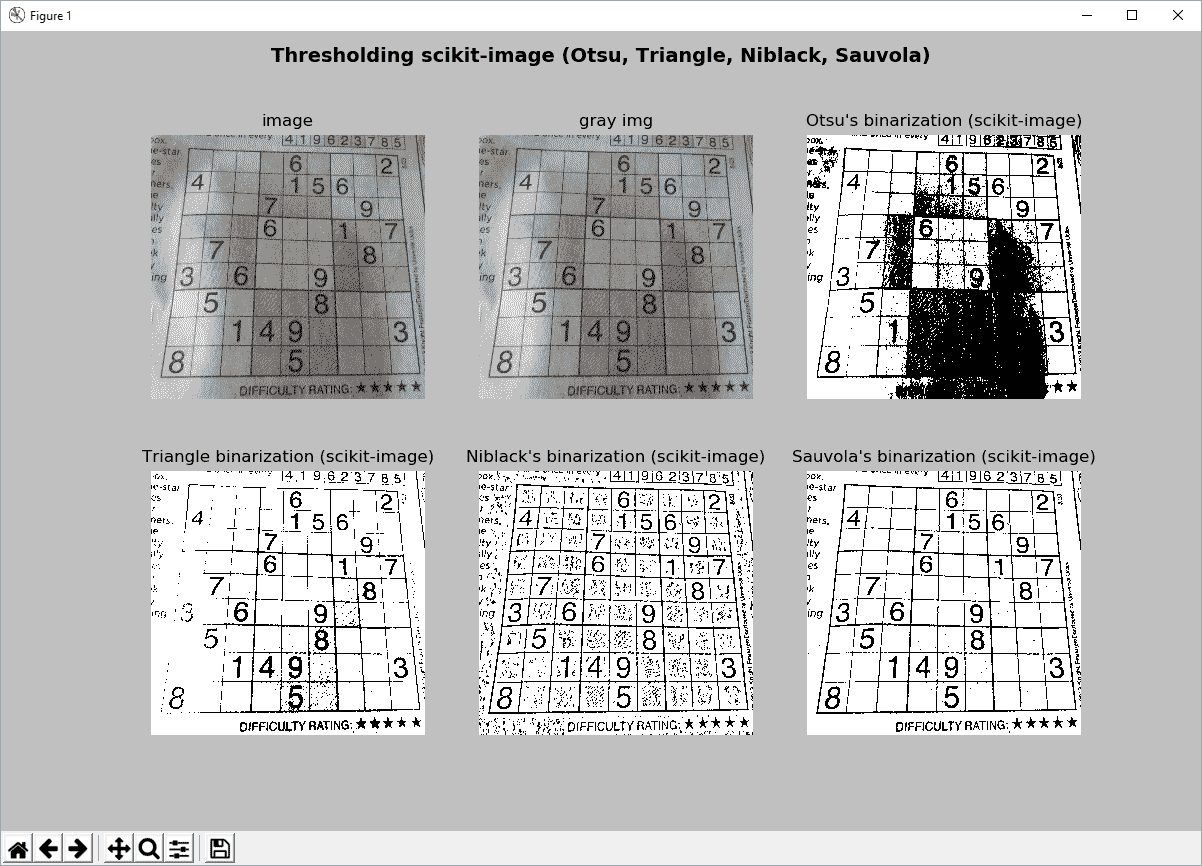# 问题

1. 使用具有阈值100cv2.threshold()cv2.THRESH_BINARY阈值类型应用阈值操作。
2. 使用cv2.adapativeThreshold()cv2.ADAPTIVE_THRESH_MEAN_CC=2blockSize=9应用自适应阈值运算。
3. 使用cv2.THRESH_BINARY阈值类型应用大津的阈值。
4. 使用cv2.THRESH_BINARY阈值类型应用三角形阈值。
5. 使用 scikit-image 应用大津的阈值。
6. 使用 scikit-image 应用三角形阈值。
7. 使用 scikit-image 应用 Niblack 的阈值。
8. 使用 scikit-image 和25的窗口大小应用 Sauvola 的阈值。
9. 修改thresholding_example.py脚本以使用np.arange()，目的是定义要应用于cv2.threshold()函数的阈值。 然后，使用定义的阈值调用cv2.threshold()函数，并将所有阈值图像存储在数组中。 最后，显示每个调用show_img_with_matplotlib()的数组中的所有图像。 将脚本重命名为thresholding_example_arange.py